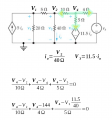# Node Voltage Method Homework

#### meatballmarinara

Joined Jan 30, 2016
1

#### bertus

Joined Apr 5, 2008
22,219

#### WBahn

Joined Mar 31, 2012
29,175
YOU need to show YOUR best attempt to solve YOUR homework problem.

First, you need to decide what analysis technique you are going to use. Usually by the time you get to this point you have node voltage analysis, mesh current analysis, and superposition in your tool box (along with the more "primitive" techniques such as direct application of KVL and KCL). Superposition doesn't apply in this case (can you explain why?). So look at the circuit and imagine the equations you will need to develop for each of the other two and decide which one you think will work best. Next, instead of charging ahead all of the way through, post your set-up equations and let's make sure they are correct before you proceed.

#### RBR1317

Joined Nov 13, 2010
709
Solving a circuit with the node voltage method presupposes that one actually has a method. So let's discuss the steps for nodal analysis. First, identify the nodes and the ground reference. Ensure that currents flowing from the nodes cover all branches. Node equations apply Kirchhoff's current law, that the algebraic sum of currents at a node must be zero. But in nodal analysis the currents are expressed in terms of node voltages, along with any given currents.

Each term in the node equation is formed as the node voltage minus the adjacent node voltage divided by the resistance to the adjacent node, for all adjacent nodes. Given currents are added directly as terms; with these conventions current flowing out of the node is considered positive. For dependent sources, write down the source definitions; use these as auxiliary equations in the general solution, or incorporate them directly into the node equations.

As an example, see the node equation for the 3 currents flowing from node V4. There will also be 3 currents at node V2, and at V1. Once the node equations are written, this ceases to be an electronics problem, and instead becomes an algebra problem.